Question

# A conical pendulum is formed by attaching a ball of mass m to a string of length L, then allowing the ball to move in a...

A conical pendulum is formed by attaching a ball of mass m to a string of length L, then allowing the ball to move in a horizontal circle of radius r. The following figure(Figure 1) shows that the string traces out the surface of a cone, hence the name.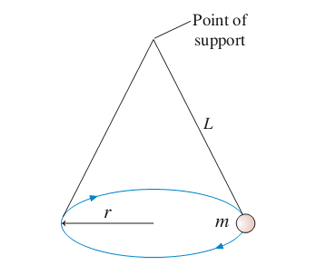Part A: Find an expression for the tension T in the string.

Express your answer in terms of the variables L,m,r and appropriate constants.

Part B: Find an expression for the ball's angular speed?.

Express your answer in terms of the variables L,r and appropriate constants.

What is the tension for a 500g ball swinging in a 20-cm-radius circle at the end of a 1.0-m-long string?

Express your answer to two significant figures and include the appropriate units.

What is angular speed (in rpm) or a 500g ball swinging in a 20-cm-radius circle at the end of a 1.0-m-long string?

Express your answer to two significant figures and include the appropriate units.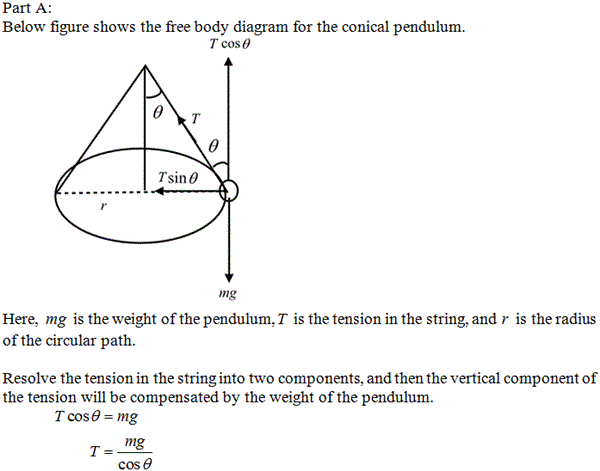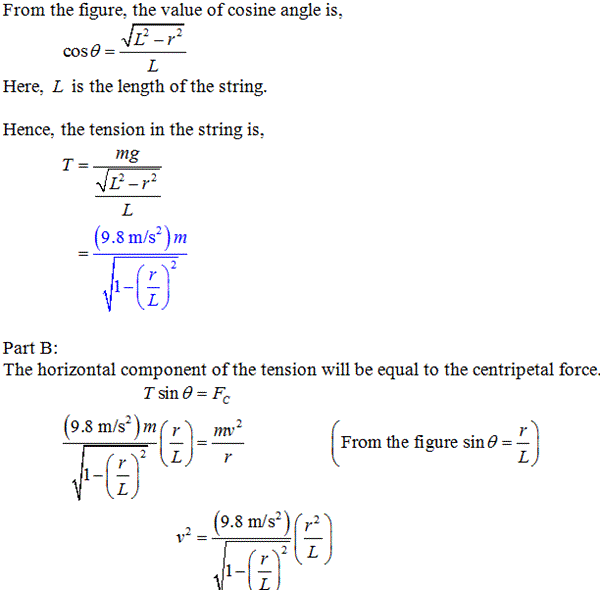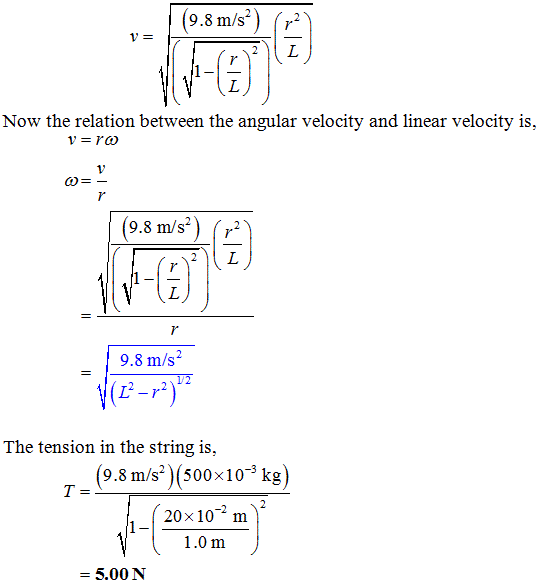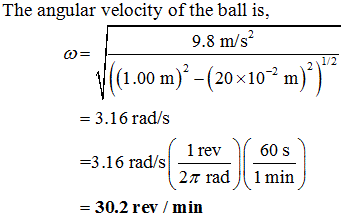#### Earn Coins

Coins can be redeemed for fabulous gifts.

Similar Homework Help Questions
• ### A conical pendulum is formed by attaching a 500g ball to a 1.0-m-long string, then allowing the...

A conical pendulum is formed by attaching a 500g ball to a 1.0-m-long string, then allowing the mass to move in a horizontal circle of a radius 20 cm the string tracesthe outside surface of the cone.a) what is the tension of the stringb) what is the ball's angular speed in rpm

• ### A conical pendulum

A conical pendulum is formed by attaching a 0.20 ball to a 1.0 -long string, then allowing the mass to move in a horizontal circle of radius 30 . The figure shows thatthe string traces out the surface of a cone, hence the name. a.) what is the tension in the string (N) b.)what is the ball's angular velocity in rpm

• ### Conical Pendulum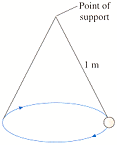A conical pendulum is formed by attaching a 400 g ball to a 1.0 m long string, then allowing themass to move in a horizontal circle of radius 30 cm. Figure P7.39 showsthat the string traces outthe surface of a cone, hence the name.Figure P7.39(a) What is the tension in the string?1 N(b) What is the ball's angular velocity, in rpm?2 rpm

• ### A conical pendulum problem where we need to solve for tension and angular speed.

A conical pendulum is formed by attaching a 500g ball to a 1m long string, then allowing the mass to move in a horizontal circle of radius 20 cm. Find the tension inthe string and the ball's angular speed in rpm's.

• ### A pendulum is constructed by attaching a mass m to an extensionless string of length l....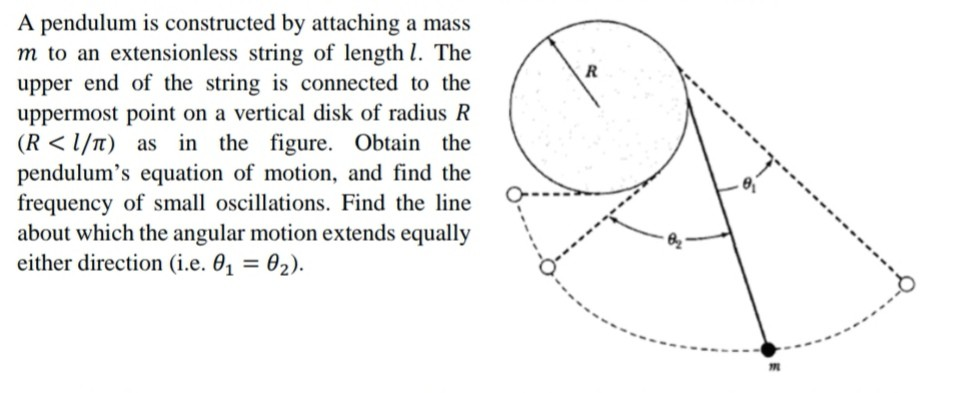A pendulum is constructed by attaching a mass m to an extensionless string of length l. The upper end of the string is connected to the uppermost point on a vertical disk of radius R (R<l/) as in the figure. Obtain the pendulum's equation of motion, and find the frequency of small oscillations. Find the line about which the angular motion extends equally either direction (i.e. 0 = 02).

• ### 3. CONICAL PENDULUM A conical pendulum is a piece of physics apparatus in which a massive...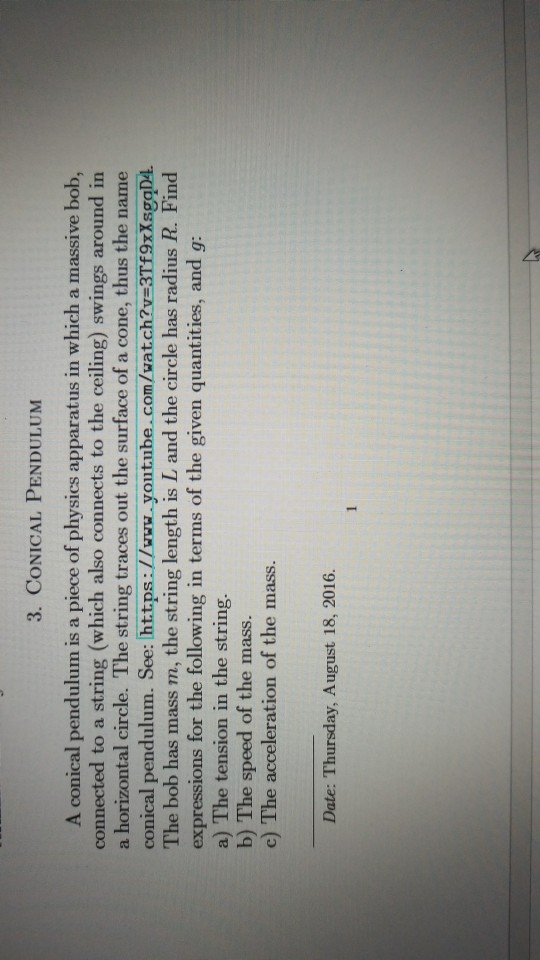3. CONICAL PENDULUM A conical pendulum is a piece of physics apparatus in which a massive bob, connected to a string (which also connects to the ceiling) swings around in a horizontal circle. The string traces out the surface of a cone, thus the name conical pendulum. See: Ihttps://www.youtube.com/watch?v 3Tf9xXsgqD4. The bob has mass m, the string length is L and the circle has radius R. Find expressions for the following in terms of the given quantities, and g a)...

• ### an ice puck of mass m revolves on an icy surface in a circle at speed...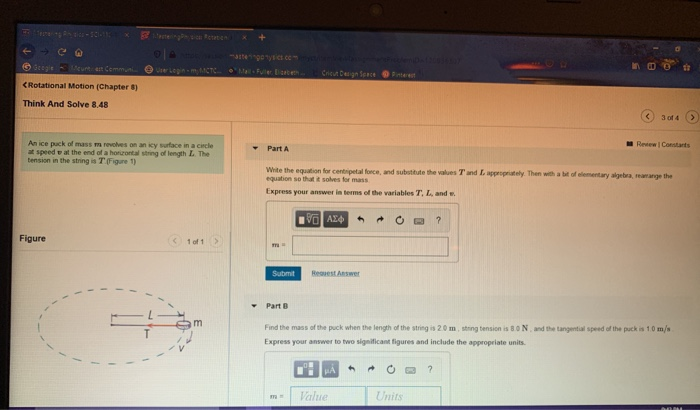an ice puck of mass m revolves on an icy surface in a circle at speed v at the end of a horizontal string of length L. the tension in the string is T. write the equation for centripetal force, and substitute the values T and L appropriately. then with a bit of elementary algebra, rearrange the equation so that it solves for mass. find the mass of the puck when the length of the string is 2.0 m, string...

• ### In this experiment, we will be swinging a block of mass m on a string of...

In this experiment, we will be swinging a block of mass m on a string of length l to study centripetal acceleration. When we use the formula a c = v 2 r to calculate the centripetal acceleration, r is the radius of the circle of the swinging block, therefore is equal to the length l of the string. Group of answer choices True or False Q2-A block of mass 0.89kg is swinging on a string. At the lowest point,...

• ### This mass (m) on a string (of length L) is moving in a horizontal circle. The...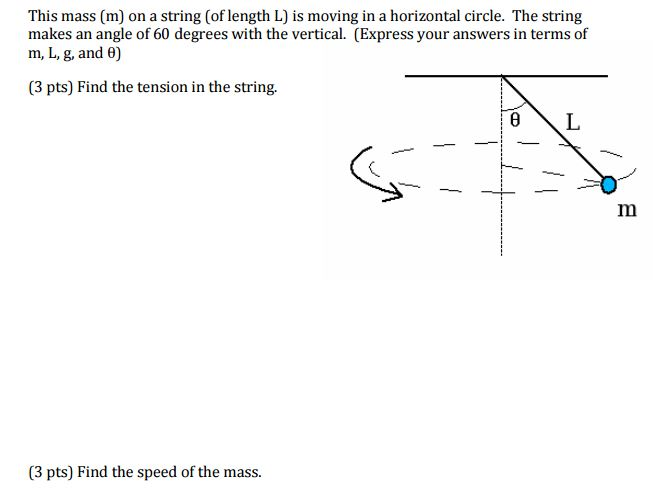This mass (m) on a string (of length L) is moving in a horizontal circle. The string makes an angle of 60 degrees with the vertical. (Express your answers in terms of m, L, g, and theta) Find the tension in the string. Find the speed of the mass.

• ### Problem# 7 On the figure below, a conical pendulum with length L- 40 cm describes a...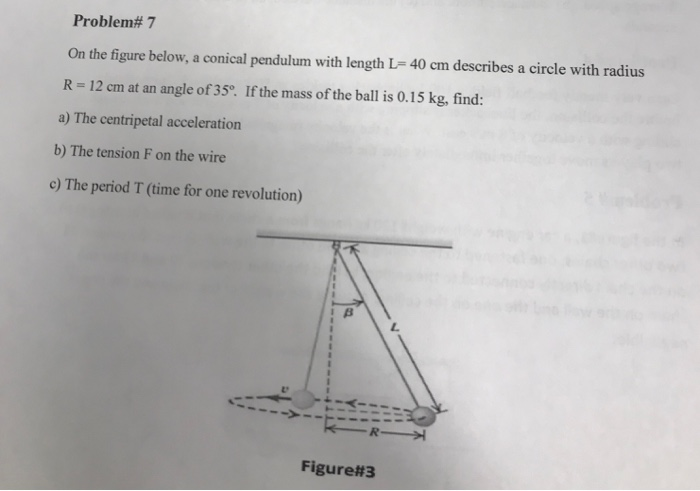Problem# 7 On the figure below, a conical pendulum with length L- 40 cm describes a circle with radius R 12 cm at an angle of 35o. If the mass of the ball is 0.15 kg, find: a) The centripetal acceleration b) The tension F on the wire c) The period T (time for one revolution) Figure#3